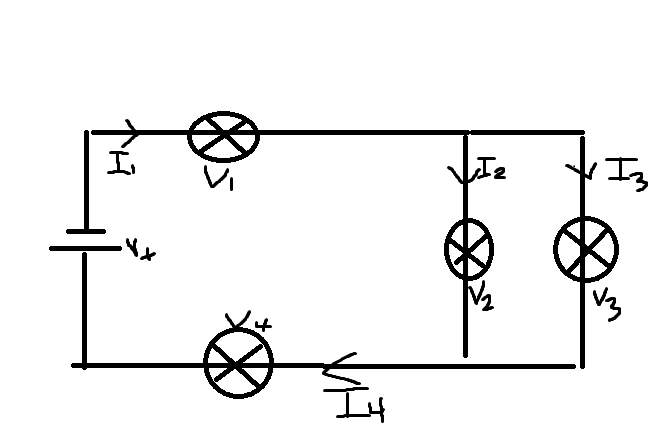# Solving for current in circuit with several light bulbs

## Homework Statement## Homework Equations

Series[/B]
Vt= V1 + V2 + V3 etc
It = I1 = I2 = I3 etc
Parallel
Vt = V1 = V2 = V3 etc
It = I1 + I2 + I3

## The Attempt at a Solution

Vt= 20V V2= 5.0 V V4= 8.0V
It= 150 mA I2= 40 mA
Find I3, and I4

I've solved for the values of V already
V1 = 7.0V
V3 = 5.0V
So Vt = V1+V2=V3+V4
= 7.0V + 5.0V + 8.0V = 20V
But I'm getting confused on solving for I which is current.

I1 is in series with I2 and I4, but I2 is also parallel to I3. So which set of laws would I apply for solving for I3?
I know I1=I4 since they're in series.
I'm assuming I1=I1=It - I2
= 150 mA - 40mA = 110mA
But still stuck on how to solve for I3, since its not stated the bulbs are identical so I'm unsure if I3 would also be 40mA. I'd appreciate any help I can receive.

Titan97
Gold Member
Shouldn't current be in the opposite direction?
Also, Have you tried applying Kirchoff's loop rule?

Shouldn't current be in the opposite direction?
Also, Have you tried applying Kirchoff's loop rule?
I copied everything from the diagram the current flows the way it does since it starts from the negative part of the cell and flows along to the positive part of the cell. I also don't believe I've learned about Kirchoff's loop rule yet. Once it reaches the box where I2 is it has two possible paths going down to V2 or proceeding forward and then down to I3/V3.

cnh1995
Homework Helper
Gold Member
I copied everything from the diagram the current flows the way it does since it starts from the negative part of the cell and flows along to the positive part of the cell. I also don't believe I've learned about Kirchoff's loop rule yet. Once it reaches the box where I2 is it has two possible paths going down to V2 or proceeding forward and then down to I3/V3.
The direction of current is conventionally from +ve terminal to -ve terminal of the battery, as Titan97 said earlier. But using the given convention, it won't affect the calculations once you flip the voltage polarities accordingly. Also, there is only one source present(Vt), rest all are bulbs.
Where is It in your diagram?

•Derpicus and Titan97
The direction of current is conventionally from +ve terminal to -ve terminal of the battery, as Titan97 said earlier. But using the given convention, it won't affect the calculations once you flip the voltage polarities accordingly. Also, there is only one source present(Vt), rest all are bulbs.
Where is It in your diagram?
Itotal is just past V4

cnh1995
Homework Helper
Gold Member
Itotal is just past V4
Then isn't It=I1? You have I1 and I2. What is the relation between i1, i2 and i3?

Then isn't It=I1? You have I1 and I2. What is the relation between i1, i2 and i3?
I know I1 is 110mA
Since I2 is 40mA
As I1=I4=It-I2
Since the total incoming has to equal the total outgoing.

But the issue I'm having is I3 is parallel to I2

Since 110ma+40 mA = 150 mA which is the It.

But the relationship for parallel is It= I1+I2 etc, so I'm unsure how to go about solving for I3.

cnh1995
Homework Helper
Gold Member
As I1=I4=It-I2
If It is just past V4, then I1=I4=It.

cnh1995
Homework Helper
Gold Member
If It is just past V4, then I1=I4=It.
KCL says incoming current at a point is equal to the outgoing current. If you apply that, you can see
I1=I2+I3.

Where are the several sources? It looks like only one. Shouldn't all V's be the same if there are no resistors?

SammyS
Staff Emeritus
Homework Helper
Gold Member
Where are the several sources? It looks like only one. Shouldn't all V's be the same if there are no resistors?
There appears to be only one source.

No. The V-s are not all the same. Several are given.

Apparently the problem statement is that:
Vt= 20V, V2 = 5.0 V, V4= 8.0V
It= 150 mA, I2= 40 mA

Find I3, and I4

And it looks like OP uses electron current, rather than conventional current..

There appears to be only one source.

No. The V-s are not all the same. Several are given.

Apparently the problem statement is that:
Vt= 20V, V2 = 5.0 V, V4= 8.0V
It= 150 mA, I2= 40 mA

Find I3, and I4

And it looks like OP uses electron current, rather than conventional current..
Thank you SammyS